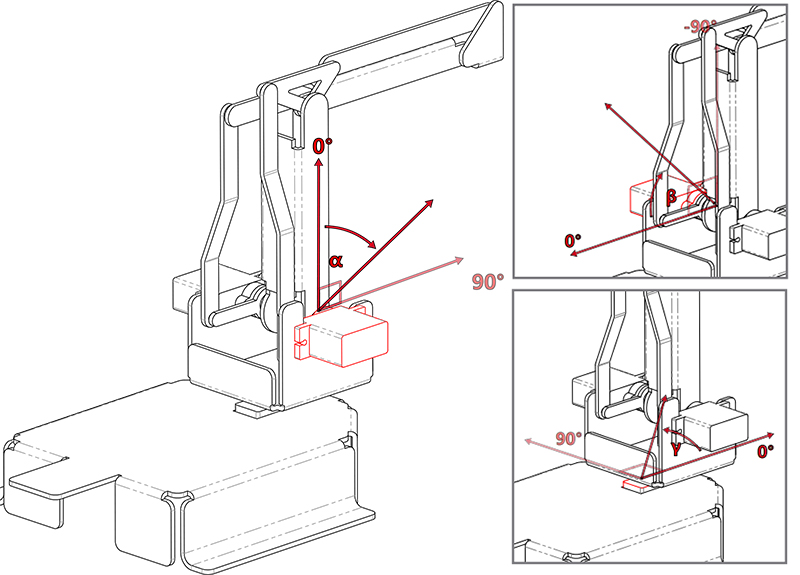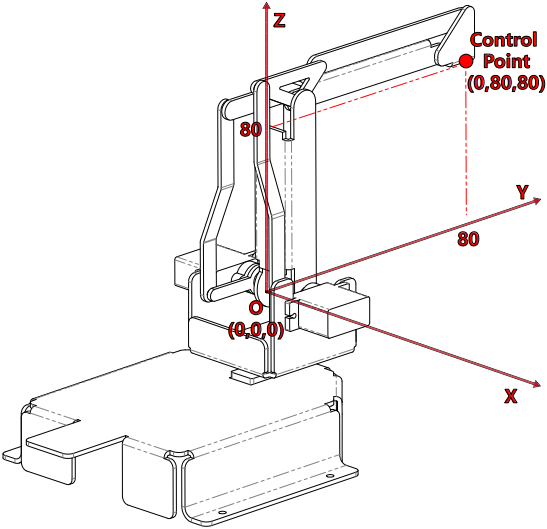# Arm¶

PiArm’s arm can be controlled in two ways: Angle Mode and Coordinate Mode.

• Angle Mode: Writes a certain angle to the three servos on the arm, thus rotating the arm to a specific position.

• Coordinate Mode: Create a spatial right-angle coordinate system for the arm and set the control point. Set the coordinates of the control point so that the arm can reach a specific position.

## Angle Mode¶

The arm has three servos to control its up and down, left and right, and front and back. We use `α`, `β` and `γ` to represent their rotation angles, as shown below.

• `α(alpha)`: represents the front-to-back rotation angle of the arm, due to the limitation of the structure, the recommended rotation range is: -30 ~ 60.

• `β(beta)`: represents the up and down rotation angle of the arm, due to the limitation of the structure, the recommended rotation range is: -60 ~ 30.

• `γ(gamma)`: represents the left and right rotation angle of the arm, the range is: -90 ~ 90.## Coordinate Mode¶

PiArm has a spatial rectangular coordinate system with its origin located at the center of the output axis of the servos on both sides. The Control Point is located at the top of the arm and is scaled in millimeters. In the initial state, the coordinates of the Control Point are (0, 80, 80).It is important to note that the arm length of PiArm is finite, and if the coordinate values are set beyond the limits of its mechanical motion, PiArm will rotate to an unpredictable position.

In other words, the total arm length of PiArm is 160 mm, which means that the limit value of the control points moving along the Y-axis should be between (0,0,0) and (0,160,0). However, due to the limitations of the structure itself, the range of movement should be much smaller than this.

• The recommended range for the X coordinate is -80 ~ 80.

• The recommended range for Y coordinate is 30 ~ 130.

• The recommended range for Z coordinate is 0 ~ 80.